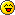# A permutation problem##A permutation problem

I had an assignment in C and I still haven't cracked a certain problem. I had to generate all combinations of 3-digit numbers from 1, 2 and 3, non-repeating. If it was repeating, it's simple. It's something like this:

Code:
`for(i=1; i<=3; i++){  for(j=1; j<=3; j++){    for(k=1; k<=3; k++){      printf("\n%d%d%d", i, j, k);    }  }}`

But my teach didn't approve. she said that I had to use 'swapping of variables' in the loop like this:
Code:
`for(i=1; i<=3; i++){  for(j=1; j<=3; j++){    for(k=1; k<=3; k++){      THE_MYSTERY_CODE    }  }}`

So I need the mystery code, which is supposed to swap vars. Any ideas?

##Re: A permutation problem

O.o I have never used C in my life...can't help you sorry

##Re: A permutation problem

It's not about C, it's about logic. Here, I'll put it in a different way:

Question: You have to generate all 3-digit numbers possible using 1, 2 and 3, without repeating anyone. So 123, 231, 132... are allowed, but 112, 222, 331 aren't allowed. So technically, there are 6 possible combinations:

123
132
213
231
312
321

All I did was put the first number constant and changed the other two numbers, I did that for 1, 2 and 3. So there are 6 possible combinations. Now, we have to use RGSS to generate, say print those 6 numbers using only loops and swapping.

Here's what swapping does:
Spoiler:

Code:
`def swap(a, b)  temp = a  a = b  b = aend`

So what that does this do? Let's say

Code:
`@one_var = 2@two_var = 6p @one_varp @two_var`

That will give two prompt windows saying '2' and '6'

Now you swap the values after declaring them and this will be the result:

Code:
`@one_var = 2@two_var = 6swap(@one_var, @two_var)p @one_varp @two_var`

Result will be '6' and '2'

So here's the template, in a manner of speaking. It's the loop function in RGSS, I think you know it:

Code:
`for i in 1 to 3  for j in 1 to 3    for k in 1 to 3      <mystery code which involves swapping of variables, but don't know WHICH variables>    end  endend`

So. I need that code inside the three loops.

##Re: A permutation problem

I'm not too sure stumped but anyway here's a guess...

Code:
`for i in 1 to 3  print iendfor j in 1 to 3swapprint jendfor k in 1 to 3swap_1print kenddef swap(1, 2, 3)  temp = 1  1 = 2  2 = 3  3 = 1enddef swap_1(1, 2, 3)  temp = 1  1 = 3  2 = 1  3 = 2end`

probably wrong...

##Re: A permutation problem

Hm, I don't get you, what does swap and swap_1 do exactly? both of them have 3 parameters. And do you mean to put the loops in one another? Even then, I don't think this would work.

I asked my teach about it and she said just one other person figured it out (GAH! And I was supposed to be best in C classes till then). I should go and ask him. But mind you, that guy has a very high IQ. In the beginning of college, there werenn't any classes, teachers just introduced themselves and soe of them gave u puzzles to solve. He solved all of them without a problem and I had a tough time cracking two of them.

##Re: A permutation problemI'm not going to uni for another 3 yrs only just 16, anyway I was too tired it was 1am to figure out how do properly

##Re: A permutation problem

lol! nvm then##Re: A permutation problem

Sponsored contentPermissions in this forum:
You cannot reply to topics in this forum